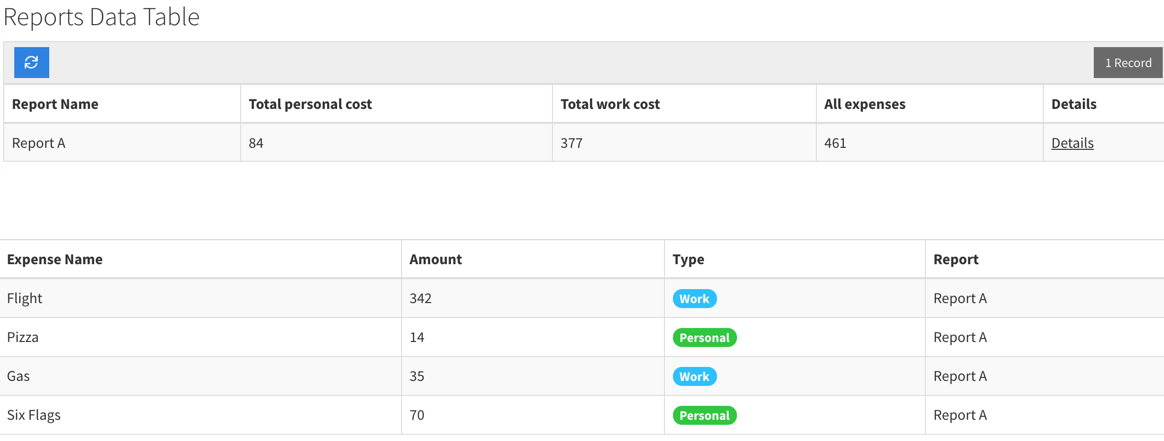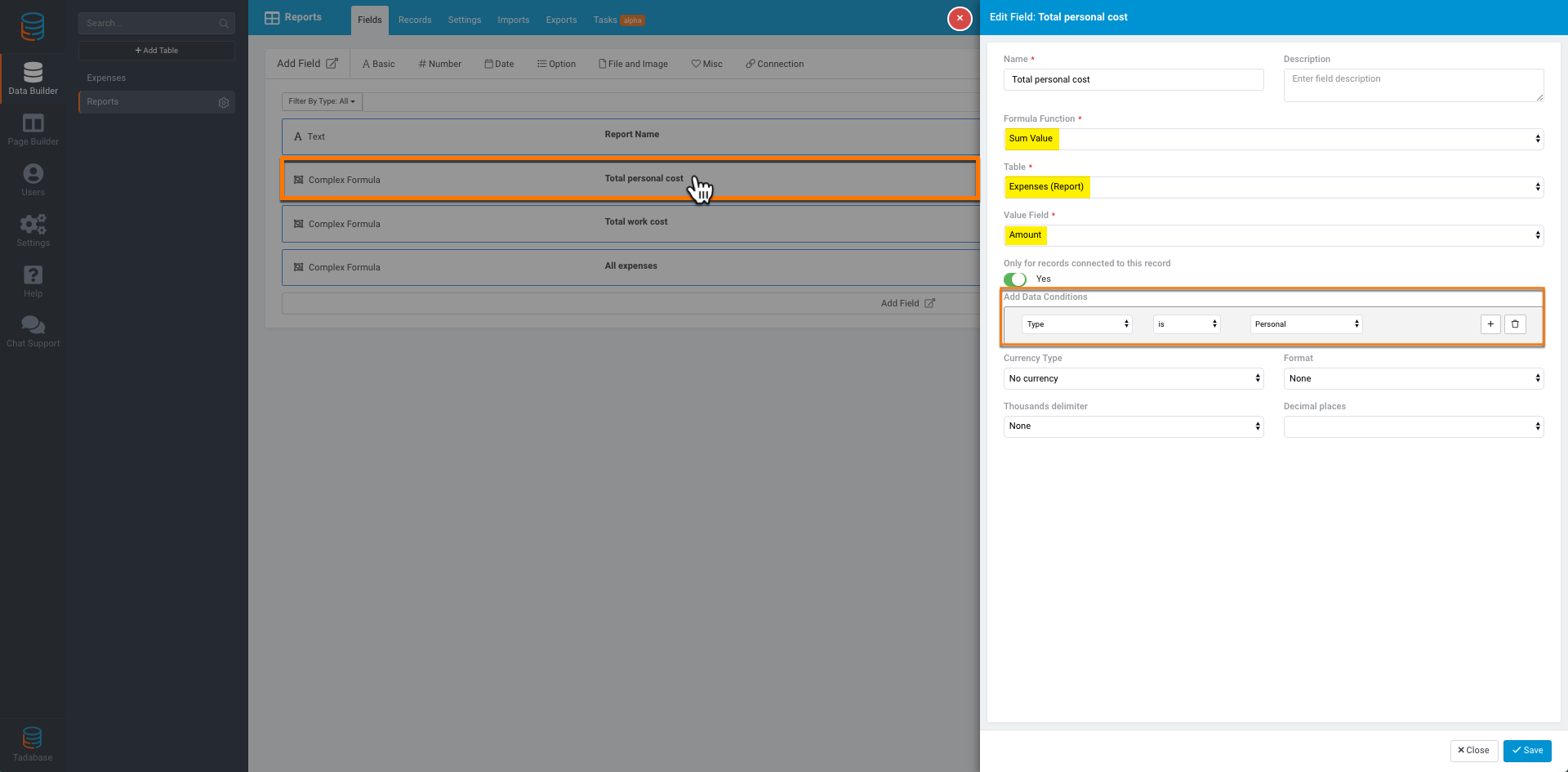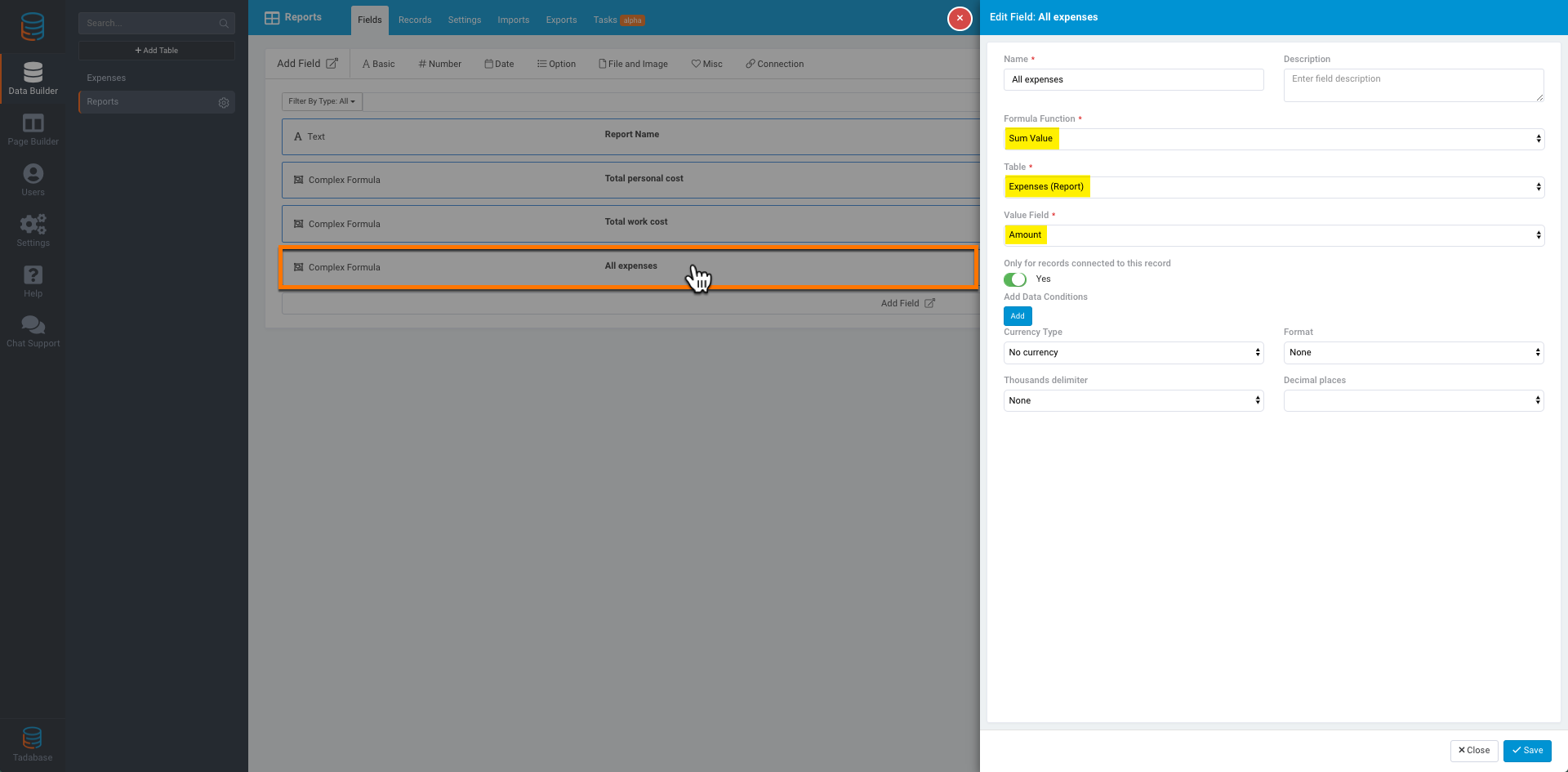# We have moved our support docs to a new location.

Using the Sum Field enables you to summarize a number field from a connected table.

This is beneficial when say, for instance, you have jobs with each job assigned to a specific user. From your Users table, you can summarize any number field in the jobs which are connected to said user.

Another example is if you have an Invoices table and an Invoice Items table. Assuming each Invoice Item belongs to a particular invoice, you'd likely want to sum all the cost fields in the Invoice Item in order to see the total from the invoice.

Another example is to have a report which will show us the sum of all the connected expenses.

Let's go through the process of using the sum field. For this example, we have two tables:

1. Expenses
2. Reports

Below is a preview of what this will look like:As you can see in the Expenses table we have:

1. Expense Name - (Text Field)
2. Amount - (Number Field)
3. Type - (Radio Field with the options "Personal" and "Work")
4. Report - (Connection field to the Reports table)

In the Reports table we have:

1. Report Name - (Text Field)
2. Total personal cost - Sum Field (Complex Formula)
3. Total work cost - Sum Field (Complex Formula)
4. All expenses - Sum Field (Complex Formula)

In the Reports table, let's take a look at each formula field to understand how it's structured.

As you can see, highlighted below we have:

• Formula Function set to Sum Value
• Table set to Expenses (Report)
• Value Field set to Amount
• Only for records connected to this record set to Yes.

Under where it says "Add Data Conditions" we've set the filter to Type is Personal so that we are only adding up the values that have been marked as Personal.

With these settings we will sum all the expenses that are connected to this report, but only where the type of expense is "Personal":For Total work cost, we have almost identical settings except that the "Add Data Conditions" is set to if Type is Work.

With these settings we will sum all the expenses that are connected to this report, but only where the type of expense is "Work":And for All expenses we have the same settings except we have no Data Condition which will sum the total without any conditions:This same type of field of structure can be used with other functions as well, such as Count total records, Min or Max